# Combustion

## Introduction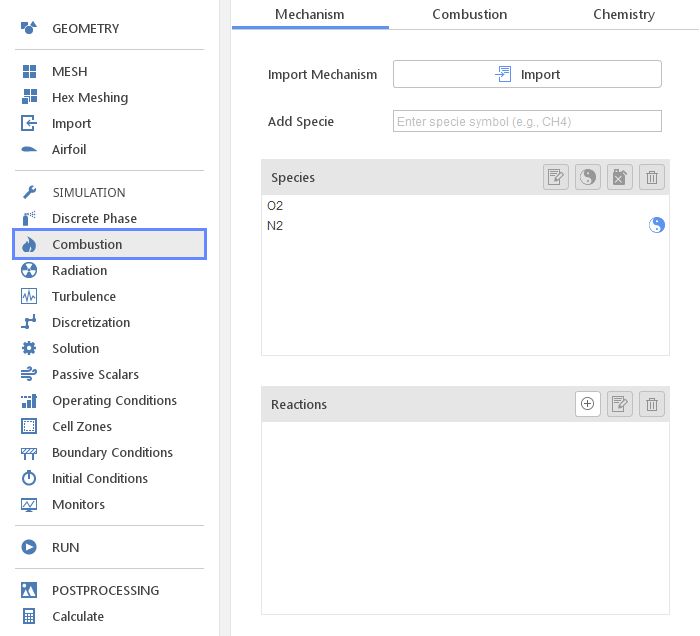The Combustion panel defines models related to species transport and combustion.

## Reaction Mechanism

In the Mechanism tab the reaction mechanism is defined. To define species transport only, the list of Species is required. To model combustion, except for Species, also Reactions are If you are interested in modeling combustion you also have to specify Reactions . You can define species and reactions manually or import entire mechanism in CHEMKIN format.

### Importing MechanismThe reaction mechanism in CHEMKIN format can be imported by clicking the Import button.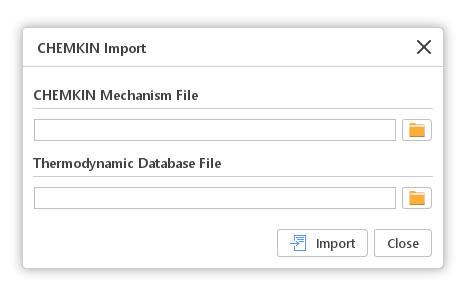After clicking the Import button, the CHEMKIN Import window will be displayed. In this window, two files are expected. The first file should contain the CHEMKIN Mechanism definition. The second file should contain Thermodynamic Database with properties for each of the species used in the mechanism. The Thermodynamic Database file is optional. If it is not specified, properties for each of the species will be taken from the built-in database.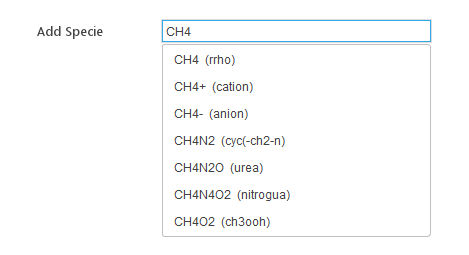If a prepared mechanism in the CHEMKIN format is not available, the species can be added from the built-in database. In the Add Specie field, the name of the specie should be given. While typing, all species whose names start with the text given, will be displayed in the list below. By clicking the specie listed, it will be added to the model.

### Species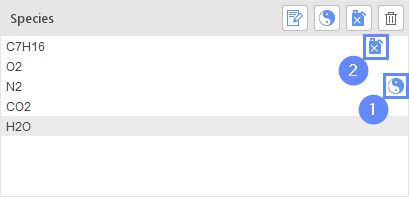In the Species list all currently used species are displayed. There are two markers that can be attached to species in the mechanism:

• Inert Specie - 1
• Fuel Specie - 2

The concentration of a specie marked as Inert will not be calculated from a transport equation. Instead, it will be set to satisfy the constraint that sum of concentrations of all species should be equal to 1.

Marking one of the species as Fuel is required by certain combustion models.

#### Editing Specie Properties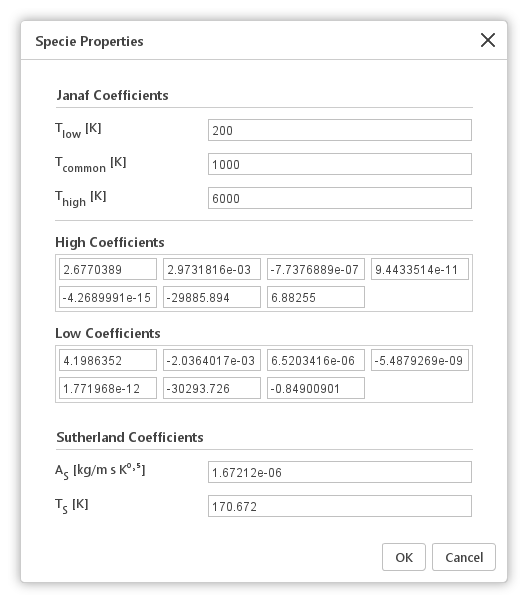Selecting a specie in the Species list and clicking the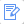Edit Species Properties a window will be displayed. In this window, he default Janaf and Sutherland coefficients of this specie can be changed.

### Reactions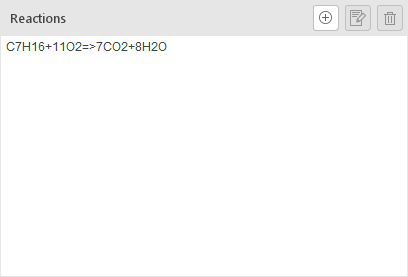The Reactions panel lists all reactions, whether imported or manually created.

## Combustion Modeling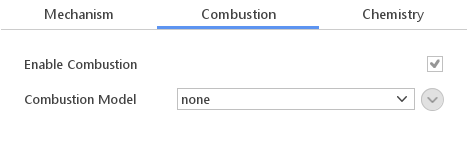The Combustion tab defines a combustion model. Optionally, using the Enable Combustion option, temporarily enables and disables combustion calculations. Disabling combustion can be beneficial during initial stages of the simulation when the flow field is far from the final solution and calculating combustion would potentially increase the convergence.

The following inputs are available in the Combustion Model:

• Infinitely Fast Chemistry
• Diffusion
• Eddy Dissipation Model
• Laminar
• Eddy Dissipation Concept
• Partially Stirred Reactor

### Infinitely Fast Chemistry Model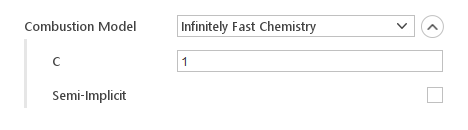Infinitely Fast Chemistry is a simple combustion model that assumes that fuel, oxidizer and products meet simultaneously and there is sufficient time to achieve equilibrium

Inputs:

• C - constant controls the distribution of the heat release over time
• Semi-Implicit - controls whether reaction rate is computed explicitly or in a semi-implicit manner

### Diffusion Combustion Model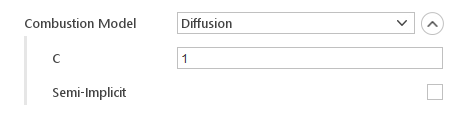Diffusion assumes that fuel and air are mixing while burning as the fire propagates and increase in size. The model is also based on single step combustion reaction, similarly to the Infinitely Fast Chemistry model.

Inputs:

• C - constant controls the distribution of the heat release over time
• Semi-Implicit - controls whether reaction rate is computed explicitly or in a semi-implicit manner

### Eddy Dissipation Model (EDM)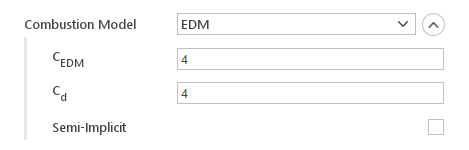Eddy Dissipation Model computes the rate of reaction under the assumption that chemical kinetics are fast compared to the rate at which reactants are mixed by turbulent eddies. The reaction rate is determined by the smallest of turbulent and diffusion time scales. Where turbulent time scale is calculated from:

$$\tau_{turb} = \frac{1}{C_{EDC}}\frac{k}{\epsilon}$$

Inputs:

• $$C_{EDM}$$ - model constant
• $$C_{d}$$ - diffusivity constant
• Semi-Implicit - controls whether reaction rate is computed explicitly or in a semi-implicit manner

### Laminar Model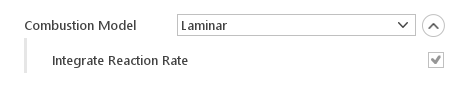Laminar model computes reaction rates directly from local thermodynamic conditions (specie concentration, temperature, pressure). It assumes no influence of turbulence on the reaction rate.

Inputs:

• Integrate Reaction Rate - specifies whether to use instantaneous or integrated reaction rates

### Eddy Dissipation Concept (EDC) turbulent combustion model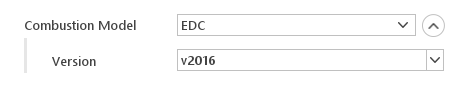This model considers that the reaction occurs in the regions of the flow where the dissipation of turbulence kinetic energy takes place (fine structures). The mass fraction of the fine structures and the mean residence time are provided by an energy cascade model.

There are many versions and developments of the EDC model, 4 of which are currently supported in this implementation: v1981, v1996, v2005 and v2016. The model variant is selected using the optional version entry in the EDC Coeffs dictionary, e.g.

### Partially Stirred Reactor Model (PaSR)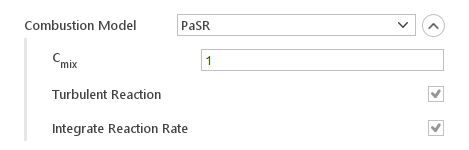The Partially Stirred Reactor model calculates reaction rate, based on both turbulence and chemistry time scales. It additionally allows for scaling the turbulent mixing time scale.

Inputs:

• $$C_{mix}$$ - coefficient for scaling turbulent time scale
• Turbulent Reaction - include turbulent effects
• Integrate Reaction Rate - specifies whether to use instantaneous or integrated reaction rates

## Chemistry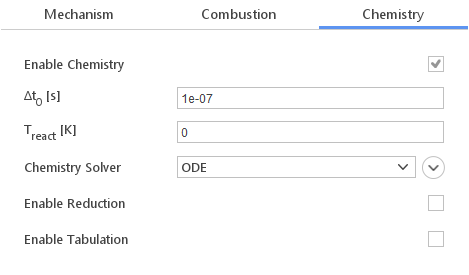In the Chemistry tab you can define how chemical reactions are calculated. You have various options to control the computational process:

• Enable Chemistry - enables or disables chemistry computation
• $$\Delta t_0 [s]$$ - initial time step for chemistry integration
• $$T_{react} [K]$$ - temperature below which chemistry is not computed
• Chemistry Solver - method for integrating reaction rates
• Enable Reduction - enables reaction mechanism reduction to reduce computational cost
• Enable Tabulation - enables tabulation of already computed reactions

### Chemistry Solver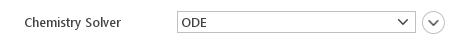The Chemistry Solver option allows you to define which technique should be used to integrate chemistry. Available chemistry solvers:

• ODE
• Implicit Euler

#### ODE Solver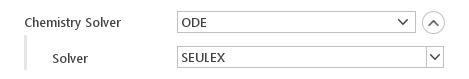The ODE solver uses ordinary differential equation (ODE) solver to integrate chemistry.

Inputs:

• Solver - ODE solver for chemistry integration

Available ODE solvers:

• SEULEUX
• RODAS - $$3^{rd}$$ and $$4^{th}$$ order
• Rosenbrock - $$2^{nd}$$, $$3^{rd}$$ and $$4^{th}$$ order
• Cash-Karp Runge-Kutta
• Dormand-Prince Runge-Kutta
• Runge-Kutta-Fehlberg
• Euler
• Euler Semi-Implicit
• Trapezoid

#### Implicit Euler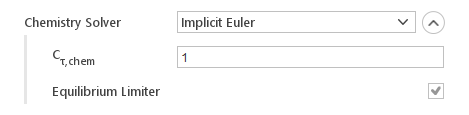The Implicit Euler uses implicit Euler technique to integrate chemistry.

Inputs:

• $$C_{\tau,chem}$$ - chemistry timescale
• Equilibrium Limiter - enables equilibrium rate limiter

### Mechanism Reduction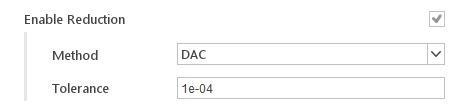Mechanism reduction option uses, instead of a full reaction mechanism, reduced mechanisms that are valid for the local thermochemical conditions.

Inputs:

• Method - mechanism reduction method
• Tolerance - tolerance for the mechanism reduction algorithm

Mechanism Reduction Methods:

• DAC - dynamic adaptive chemistry
• DRG - directed relation graph
• DRGEP - directed relation graph with error propagation
• PFA - path flux analysis
• EFA - element flux analysis

### Chemistry Tabulation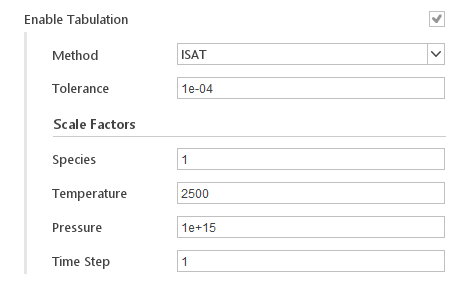Chemistry tabulation technique intends to reuse computationally demanding results, e.g. the integration of large and stiff ODE systems, by storing those results and all the data needed to retrieve them.

Inputs:

• Method - tabulation method, currently only in-situ adaptive tabulation (ISAT) is available
• Tolerance - tolerance for retrieving data from table instead of computing them directly
• Species - scale factor for species
• Temperature - scale factor for temperature
• Pressure - scale factor for pressure
• Time Step - scale factor for time step